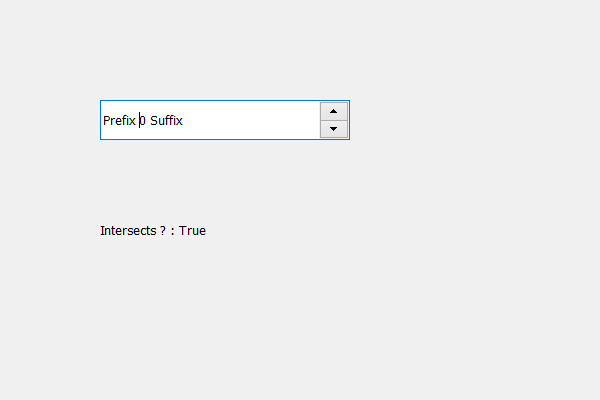Skip to content
Related Articles
PyQt5 QSpinBox – Checking if certain region intersects the Children region
• Last Updated : 19 May, 2020

In this article we will see how we can check if a certain region it can be QRect or QRegion intersects children region of spin box, children region holds the combined region occupied by the spin box’s children. In order to get the children region we use `childrenRegion` method.

In order to do this we use intersects method with the children region object of the spin box

Syntax : children_region.intersects(rectangle)

Argument : It takes QRect object as argument also it can take QRegion object

Return : It returns bool

Below is the implementation

 `# importing libraries``from` `PyQt5.QtWidgets ``import` `*` `from` `PyQt5 ``import` `QtCore, QtGui``from` `PyQt5.QtGui ``import` `*` `from` `PyQt5.QtCore ``import` `*` `import` `sys`` ` ` ` `class` `Window(QMainWindow):`` ` `    ``def` `__init__(``self``):``        ``super``().__init__()`` ` `        ``# setting title``        ``self``.setWindowTitle(``"Python "``)`` ` `        ``# setting geometry``        ``self``.setGeometry(``100``, ``100``, ``600``, ``400``)`` ` `        ``# calling method``        ``self``.UiComponents()`` ` `        ``# showing all the widgets``        ``self``.show()`` ` `        ``# method for widgets``    ``def` `UiComponents(``self``):``        ``# creating spin box``        ``self``.spin ``=` `QSpinBox(``self``)`` ` `        ``# setting geometry to spin box``        ``self``.spin.setGeometry(``100``, ``100``, ``250``, ``40``)`` ` `        ``# setting range to the spin box``        ``self``.spin.setRange(``0``, ``999999``)`` ` `        ``# setting prefix to spin``        ``self``.spin.setPrefix(``"Prefix "``)`` ` `        ``# setting suffix to spin``        ``self``.spin.setSuffix(``" Suffix"``)`` ` `        ``# getting the children region``        ``children_region ``=` `self``.spin.childrenRegion()`` ` `        ``# creating a label``        ``label ``=` `QLabel(``self``)``        ``label.setWordWrap(``True``)`` ` `        ``# setting geometry to the label``        ``label.setGeometry(``100``, ``200``, ``200``, ``60``)`` ` `        ``# QRect``        ``rectangle ``=` `QRect(``1``, ``1``, ``20``, ``30``)`` ` `        ``# checking if rectangle intersects the children region``        ``check ``=` `children_region.intersects(rectangle)`` ` `        ``# setting text to the label``        ``label.setText(``"Intersects ? : "` `+` `str``(check))`` ` ` ` ` ` `# create pyqt5 app``App ``=` `QApplication(sys.argv)`` ` `# create the instance of our Window``window ``=` `Window()``window.spin.setFocus()``# start the app``sys.exit(App.``exec``())`

Output :Attention geek! Strengthen your foundations with the Python Programming Foundation Course and learn the basics.

To begin with, your interview preparations Enhance your Data Structures concepts with the Python DS Course. And to begin with your Machine Learning Journey, join the Machine Learning – Basic Level Course

My Personal Notes arrow_drop_up